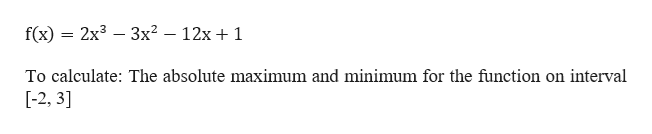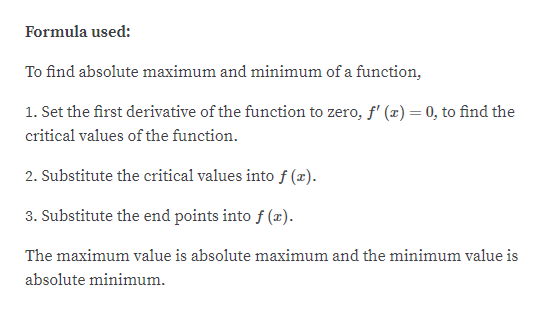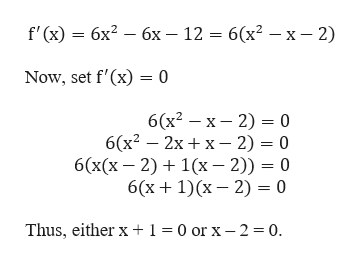# Can you show me the steps to solve the problem?3.1Directions: Find the absolute maximum and absolute minimum values of f on the given interval.47) f(x) = 2x3 - 3x2 - 12x + 1 [-2, 3]

Question
32 views

Can you show me the steps to solve the problem?

3.1

Directions: Find the absolute maximum and absolute minimum values of f on the given interval.

47) f(x) = 2x3 - 3x2 - 12x + 1 [-2, 3]

check_circle

Step 1

Given a function and an interval,help_outlineImage Transcriptionclose2x33x212x 1 f(x) = To calculate: The absolute maximum and minimum for the function on interval [-2, 3] fullscreen
Step 2

Note:help_outlineImage TranscriptioncloseFormula used: To find absolute maximum and minimum of a function, 1. Set the first derivative of the function to zero, f' (a)= 0, to find the critical values of the function 2. Substitute the critical values intof (x) 3. Substitute the end points into f (x) The maximum value is absolute maximum and the minimum value is absolute minimum fullscreen
Step 3

Begin with calculating the first derivative of...help_outlineImage Transcriptionclosef' (x) 6x 6x - 12 6x2 - x - 2) Now, set f'(x) 0 6(x2 x 2) = 0 6(x2 2x x- 2) 0 6(x(x 2)1x 2)) 0 6(x 1)(x 2) = 0 Thus, either x +1 0 or x - 2 0. fullscreen

### Want to see the full answer?

See Solution

#### Want to see this answer and more?

Solutions are written by subject experts who are available 24/7. Questions are typically answered within 1 hour.*

See Solution
*Response times may vary by subject and question.
Tagged in

### Calculus